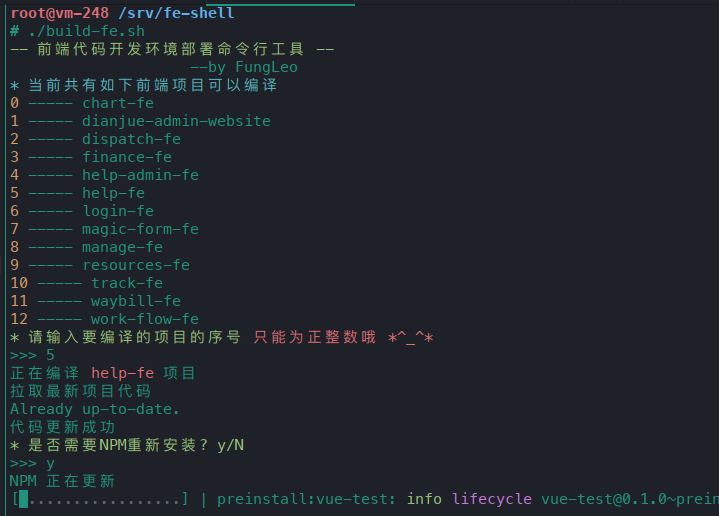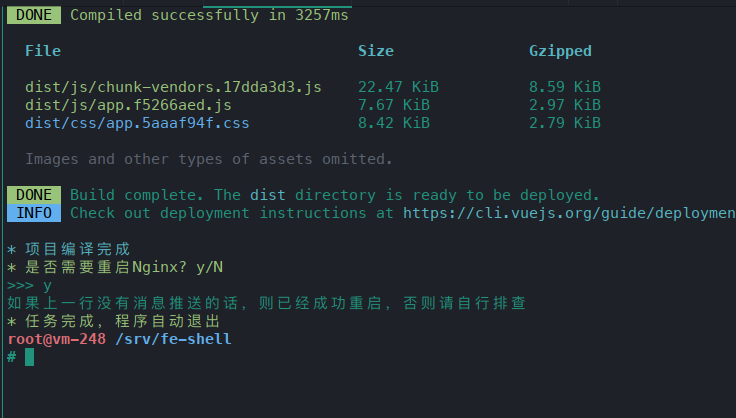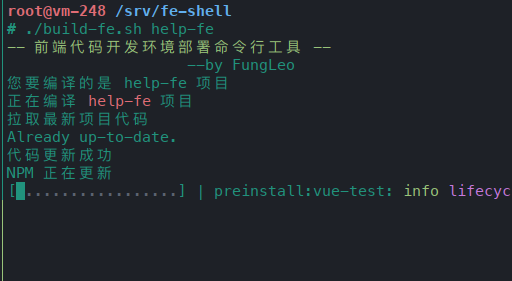# 一段自动编译前端项目的Shell代码，如果没有上集成工具的话，略有小用。#!/bin/bash

upNpm () {
echo "NPM 正在更新"
npm i
echo "NPM 更新完成"
git checkout package-lock.json
}

echo "如果上一行没有消息推送的话，则已经成功重启，否则请自行排查"
}

dir=/srv/web-fe/
echo -e "\033[32m-- 前端代码开发环境部署命令行工具 --\033[0m"
echo "                    --by FungLeo"
if [ "x$1" != "x" ];then echo "您要编译的是$1 项目"
project=$1 else echo -e "\033[36m* 当前共有如下前端项目可以编译\033[0m" arr=($(ls $dir)) i=0 for s in${arr[@]};do
echo -e "\033[33m$i\033[0m -----$s"
let i+=1
done
echo -e "\033[32m* 请输入要编译的项目的序号 \033[31m只能为正整数哦 *^_^*\033[0m"

length=${#arr[@]} if [$index -ge $length ]; then echo -e "\033[31m* 输入的数字大于项目总数 >_< \033[0m程序自动退出" exit fi project=${arr[index]}
fi
doPro=$dir$project
if [ ! -d "$doPro" ];then echo -e "\033[31m 您要部署的项目不存在，程序自动退出 \033[0m" exit fi echo -e "正在编译\033[31m$project \033[0m项目"
cd $doPro echo "拉取最新项目代码" git pull echo '代码更新成功' if [ "x$1" != "x" ];then
upNpm
else
echo -e "\033[32m* 是否需要NPM重新安装? y/N\033[0m"
if [ "$yn" == "Y" -o "$yn" == "y" ]; then
upNpm
fi
fi
echo -e '\033[36m* 项目开始编译\033[0m'
npm run build
echo -e '\033[36m* 项目编译完成\033[0m'
if [ "x$1" != "x" ];then reloadNginx else echo -e "\033[32m* 是否需要重启Nginx? y/N\033[0m" read -p ">>> " yn if [ "$yn" == "Y" -o "\$yn" == "y" ]; then
fi
fi
echo -e '\033[32m* 任务完成，程序自动退出\033[0m'
exit


©️2019 CSDN 皮肤主题: 编程工作室 设计师: CSDN官方博客